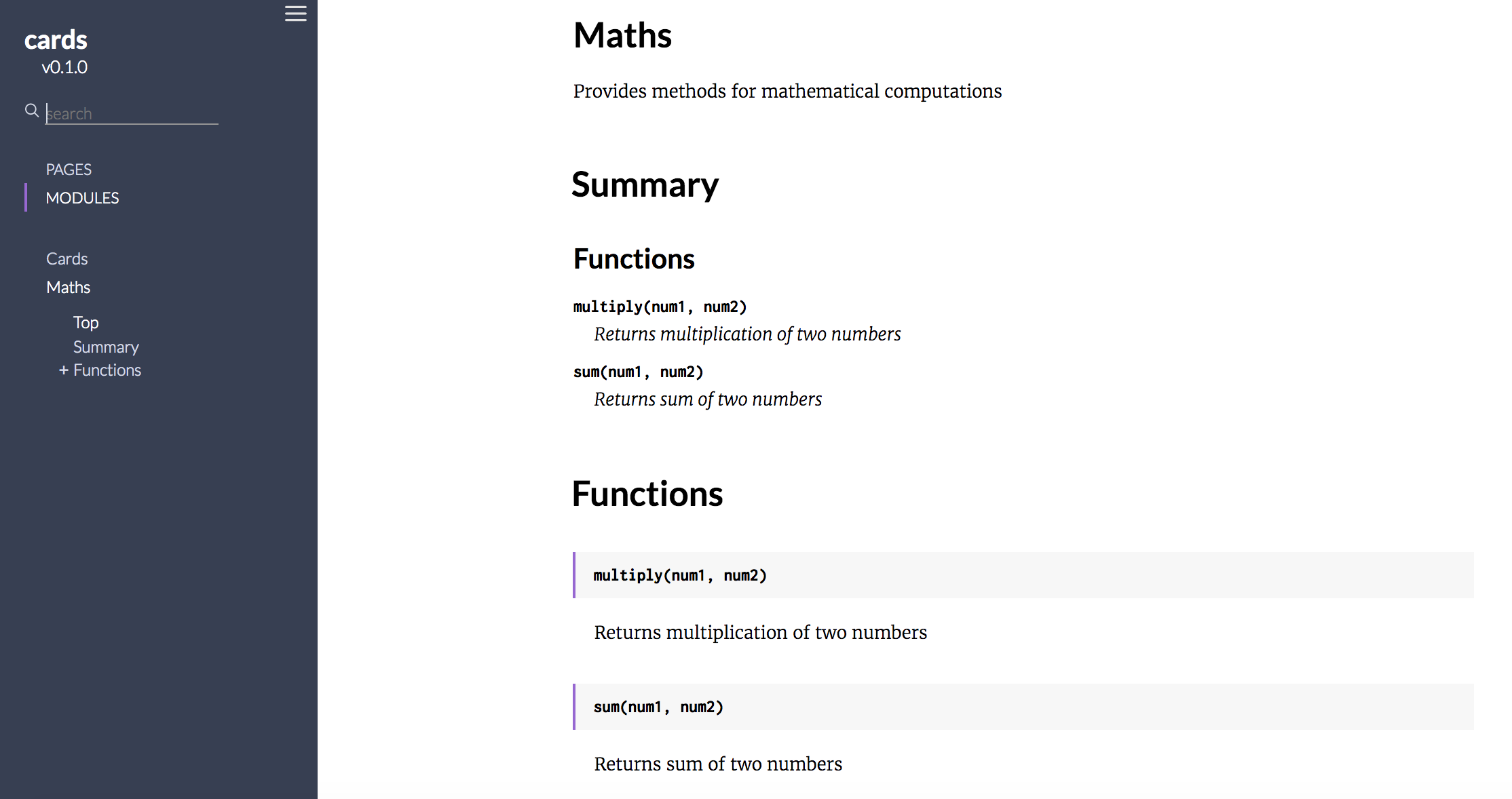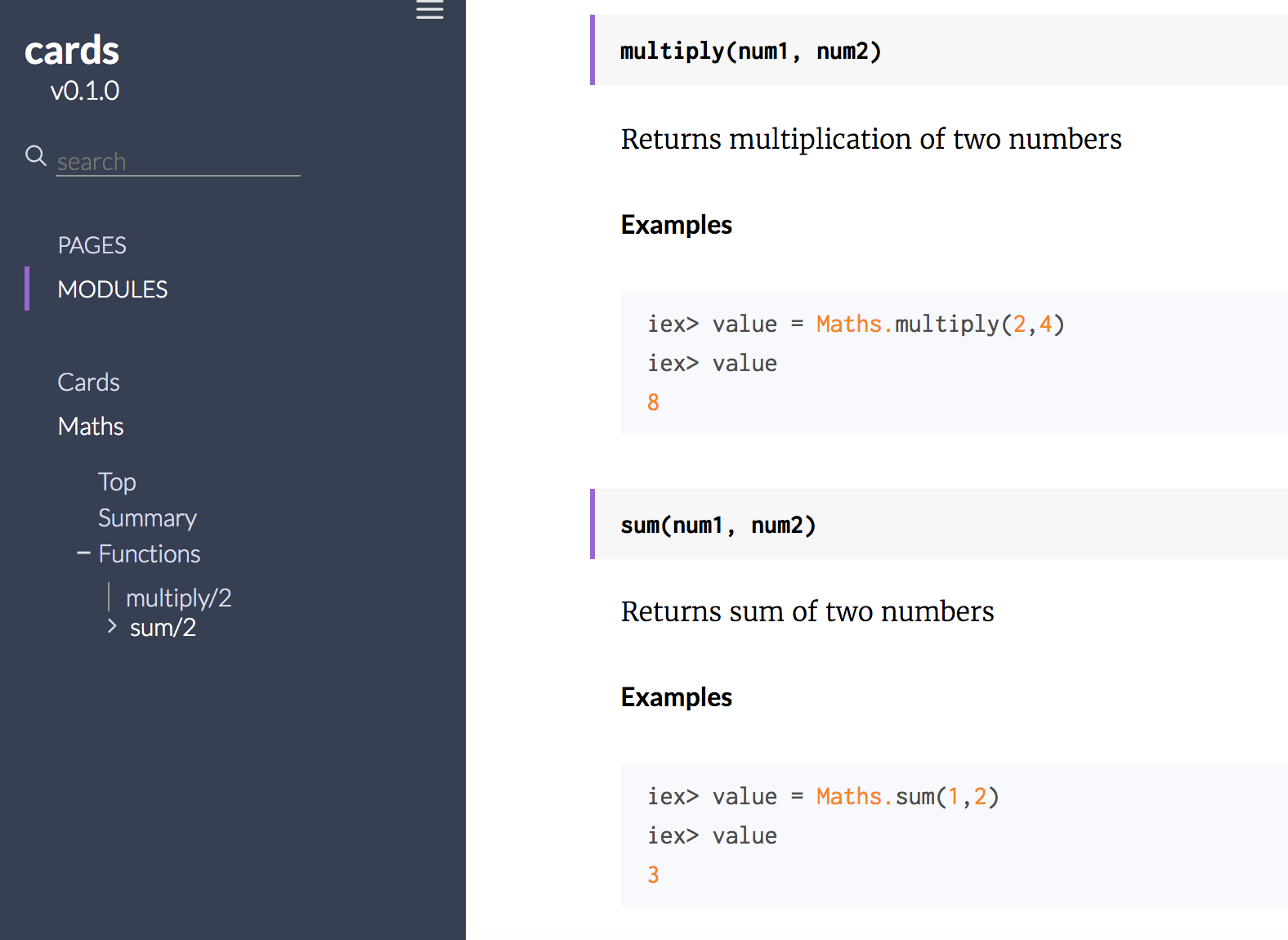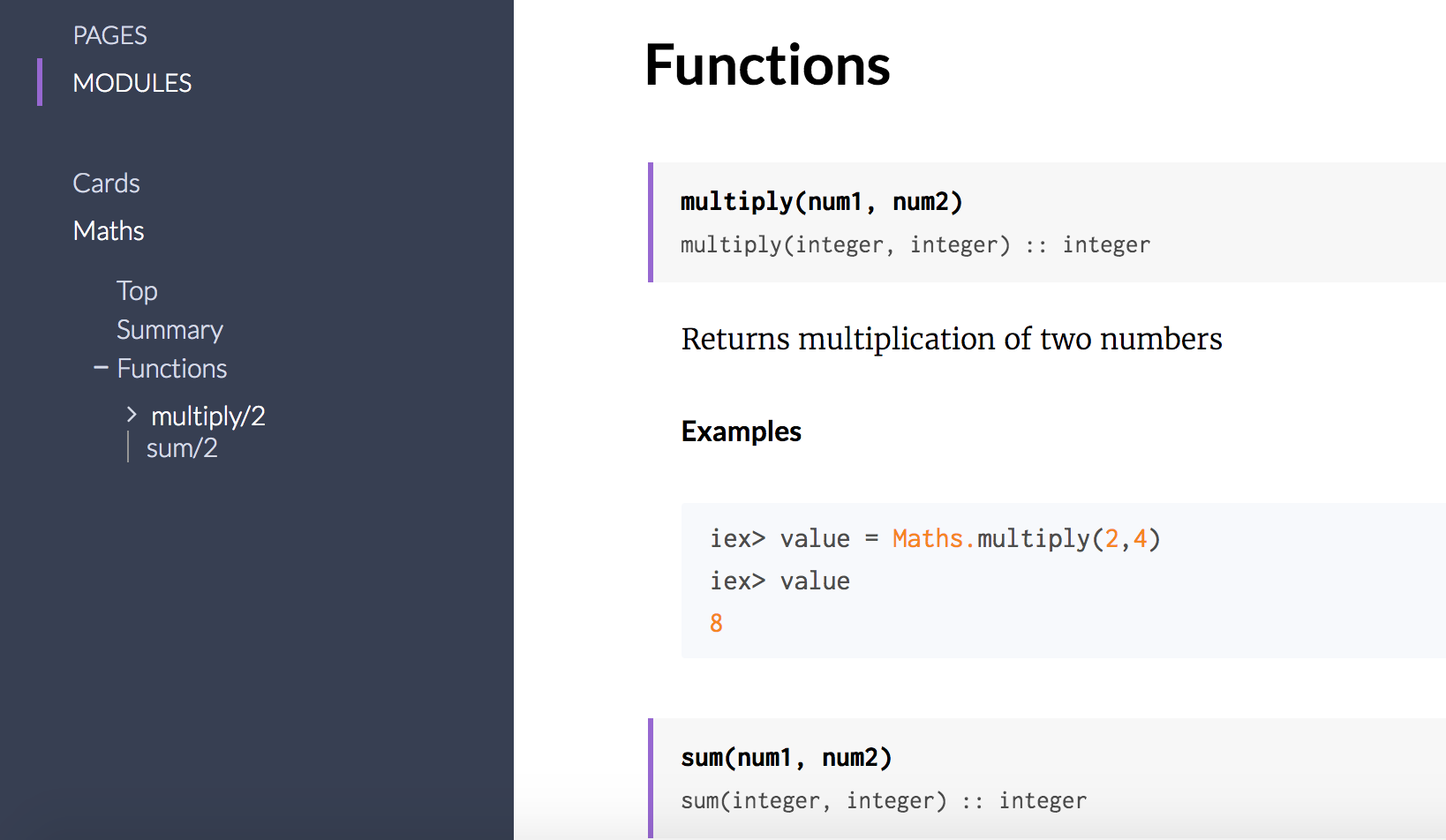One of the coolest feature I came across in Elixir - our code documentation becomes our unit tests. I was amazed by its simplicity when I saw it in action in sample app I was writing. I feel this is one of the most incredibly and helpful feature I saw in any modern programming language in recent times. (Python has similar functionality using Python’s doctest.)

Let me explain this by giving a quick demo using few simple examples: Math functions sum and multiply.

Lets say this is our `Maths` module

``````defmodule Maths do
def sum(num1, num2) do
num1 + num2
end

def multiply(num1, num2) do
num1 * num2
end
end
``````

First, lets add simple documentation to this `Maths` module and its functions `sum` and `multiply`

``````defmodule Maths do

@moduledoc """
Provides functions for mathematical computations
"""

@doc """
Returns sum of two numbers
"""
def sum(num1, num2) do
num1 + num2
end

@doc """
Returns multiplication of two numbers
"""
def multiply(num1, num2) do
num1 * num2
end

end
``````

To generate documentation for this module and its functions, we need to run command (on console) `mix docs` on our Elixir project root.

This will generate following files and outcome

• Docs successfully generated.
• View them at "doc/index.html".

NOTE: If you face any error while running command `mix docs` then verify if your project `mix.exs` file has hex packages `ex_doc` and `earmark` present. If not, then we will need to add following 2 lines

``````{:earmark, "~> 0.1", only: :dev},
{:ex_doc, "~> 0.11", only: :dev}
``````

Opening file `/doc/index.html` shows following nice documentation created by Elixir out of the box. Very neat.Now we will write few examples in our documentation for functions `sum` and `multiply` and they will become tests. First, lets run these two functions in our console using `iex -S mix`

``````iex(9)> value = Maths.sum(1,2)
3
iex(10)> value
3
iex(11)>

iex(11)> value = Maths.multiply(2,4)
8
iex(12)> value
8
iex(13)>
``````

We will copy paste same examples in our documentation now (three tabs indentation inside Examples block is important below.)

``````  @doc """
Returns sum of two numbers

## Examples
iex> value = Maths.sum(1,2)
iex> value
3
"""
def sum(num1, num2) do
num1 + num2
end

@doc """
Returns multiplication of two numbers

## Examples
iex> value = Maths.multiply(2,4)
iex> value
8
"""
def multiply(num1, num2) do
num1 * num2
end
``````

Generate documentation again using command `mix docs` and it will now look nicely formatted as shown in following screenshot.These documentation examples will lead to some very interesting behaviour. Testing in Elixir is first class citizen i.e. unit testing is fully featured out of the box in Elixir without a real need to add any additional plugins or libraries to write and run tests around our code. Elixir’s built-in test framework is `ExUnit` and it includes everything we need to thoroughly test our code. And its the `ExUnit.DocTest` that allows us to generate tests from the code examples.

So the real magic starts now, lets run our tests and see this in live action. Following command on console will run our example tests from documentation

``````mix test
``````

This will now show outcome as

``````Compiling 1 file (.ex)
..

Finished in 0.03 seconds
2 tests, 0 failures
``````

Lets attempt to make our tests fail by writing incorrect outcome for `sum` function i.e. lets change the Example snippet for `sum` to

``````## Examples
iex> value = Maths.sum(1,2)
iex> value
5
``````

And lets run command `mix test` again. This time the output is

``````Compiling 1 file (.ex)
.

1) test doc at Maths.sum/2 (2) (MathsTest)
test/maths_test.exs:3
Doctest failed
code: value = Maths.sum(1,2)
value === 5
lhs:  3
stacktrace:
lib/maths.ex:11: Maths (module)

Finished in 0.05 seconds
2 tests, 1 failure
``````

So the output clearly says its expecting value `3` whereas it got value as `5`. Lets fix it and run again.

``````## Examples
iex> value = Maths.sum(1,2)
iex> value
3
``````

And lets run command `mix test` again. This time there will be 0 failures.

``````Compiling 1 file (.ex)
..

Finished in 0.03 seconds
2 tests, 0 failures
``````

Another attribute we can add to our functions is `@spec`, which then allows us to define the types that the inputs and outputs of the function will take. It then gets added to pages i.e. documentation created by ExDoc and allows us to get a better understanding of how to use the function. For example:

``````@spec multiply(integer, integer) :: integer
def multiply(num1, num2) do
num1 * num2
end
``````

This will show up in our documentation as follows:Further reading and references on DocTesting:
DocTests in Elixir

Thanks to josevalim and milmazz, owners and maintainers of ExDoc.